# PSEB 10th Class Science Solutions Chapter 12 Electricity

Punjab State Board PSEB 10th Class Science Book Solutions Chapter 12 Electricity Textbook Exercise Questions and Answers.

## PSEB Solutions for Class 10 Science Chapter 12 Electricity

PSEB 10th Class Science Guide Light Reflection and Refraction Textbook Questions and Answers

Question 1.
A piece of wire of resistance R is cut into five equal parts. These parts are then connected in parallel. If the equivalent resistance of this combination is R’, then the ratio R/R’ is
(a) 1/25
(b) 1/5
(c) 5
(d) 25.
On cutting wire of resistance R into five equal parts, the resistance of each part is $$\frac{R}{5}$$. Let the total resistance be R’ of 5 resistors each of resistance R/5 connected in parallel.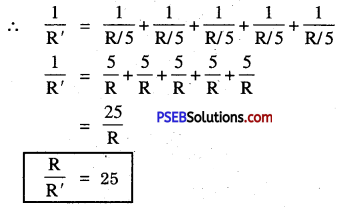Hence answer (d) 25 is correct.

Question 2.
Which of the following terms does not represent electrical power in the circuit?
(a) I2R
(b) IR2
(c) VI
(d) V2/R
All three (a), (c) and (d) represent power. Therefore, only (b) IR2 does not represent power.

Question 3.
An electric bulb is rated 220 V and 100 W. When operated on 110 V, the power consumed will be
(a) 100 W
(b) 75 W
(c) 50 W
(d) 25 W.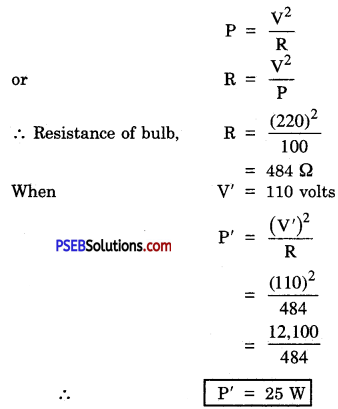Hence (d) 25 W is correct answer.

Question 4.
Two conducting wires of the same material and of equal lengths and equal diameters are first connected in series and then in parallel in an electric circuit. The ratio of heat produced in series and parallel combination would be
(a) 1 : 2
(b) 2 :1
(c) 1: 4
(d) 4 : 1
Since the wires are of the same material and are equal in lengths and diameters, therefore, if R is resistance of each. Let total resistance in series and parallel be Rs and R respectively, when connected in series ;
Rs = R + R
= 2R
When connected to a source V, then
Heat produced in series Hs = $$\frac{\mathrm{V}^{2} t}{\mathrm{R}_{s}}$$
when connected is parallel, $$\frac{1}{\mathrm{R}_{p}}=\frac{1}{\mathrm{R}}+\frac{1}{\mathrm{R}}$$
$$\frac{2}{R}$$
or
Rp = R/2
Heat produced in parallel Hp = $$\frac{\mathrm{V}^{2} t}{\mathrm{R}_{p}}$$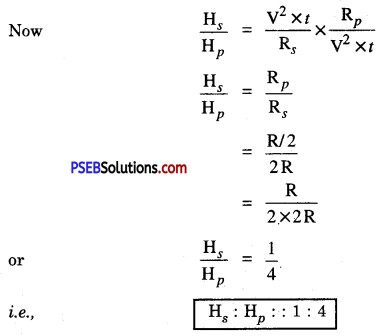Hence, (c) 1 : 4 is correct answer.

Question 5.
How is a voltmeter connected in the circuit to measure potential difference between two points?
Voltmeter is always connected in parallel between two points across which the potential difference is required to be measured.Question 6.
A copper wire has diameter 0.5 mm and resistivity 1.6 × 10-8 Ω m. What will be the length of this wire to make its resistance 10 Ω? How much does the resistance change if the diameter is doubled?
Here, Diameter (d) = 0.5 mm
= 0.5 × 10-3 m
= 5 × 10-4 m
Resistivity
ρ = 1.6 × 10-8 Ω m
l = 1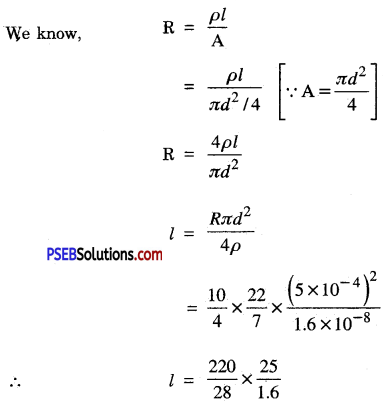l = 122.76 m
If the diameter of wire is doubled, resistance will become l/4th of the original resistance, since R ∝ 1/A ,
or R ∝ l2d2
Now Resistance $$\frac{R}{4}=\frac{10}{4}$$.
= 2.5 Ω
Decrease in resistance will be = 10 – 10/4
= 10 – 2.5
= 7.5 ohm

Question 7.
The values of the current I flowing in a given resistor for corresponding values of potential difference V across the resistor are given below :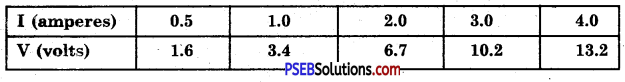Plot a graph between V and I and calculate the resistance of that resistor.
Graph between I and V is as shown in Figure It is almost a straight line.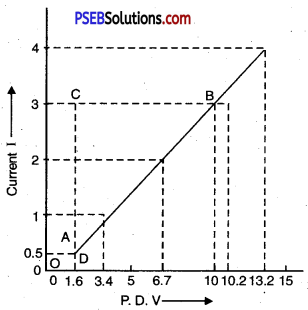Resistance is the slope of I-V graph.
R = Slope = $$\frac{B C}{A C}$$
or R = $$\frac{10.0-1.6}{3-0.5}$$
= $$\frac{8.4}{2.5}$$
= $$\frac{84}{25}$$ = 3.36 Ω
∴ R = 3,36 Ω

Question 8.
When a 12 V battery is connected across an unknown resistor, there is a current of 2.5 mA in the circuit. Find the value of the resistance of resistor?
Here, I = 2:5 mA = 2.5 × 10-3 A; V = 12 volts ; R =?
We know from ohm’s law
V = IR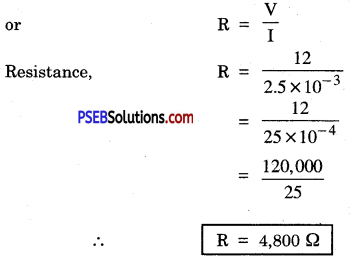Question 9.
A battery of 9 V is connected in series with resistors of 0.2 Ω, 0.3 Ω, 0.4 Ω, 0.5 Ω and 12 Ω respectively. How much current will flow through a 12 Ω resistor?
Resistors are connected in series
∴ Total Resistance, R8 = 0.2 + 0.3 + 0.4 + 0.5 + 12 = 13.4 Ω
Potential Difference V = 9 V
Resistance, R = $$\frac{V}{I}$$
or I = $$\frac{V}{R}$$
= $$\frac{9}{13.4}$$
or I = 0.67 A
When in series, the current passing through each resistor is equal to the current in the circuit.
∴ Current through 12 Ω = 0.67 A

Question 10.
How many 176 Ω resistors in parallel are required to carry 5A on a 220 V line?
Here R = 176 Ω
V= 220V
I = 5A
Resistance of parallel combination Rp = $$\frac{V}{I}$$
Rp = $$\frac{220}{5}$$

If n resistors each of resistance R are connected in parallel, then net resistance Rp is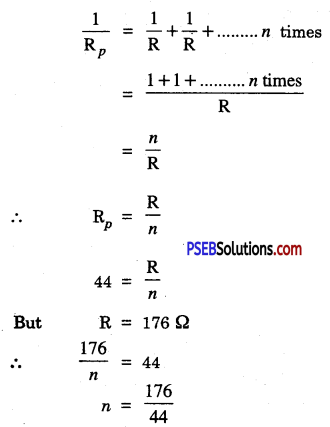or n = 4
4 resistors each 176 Ω connected in parallel will result in net resistance of 44 Ω causing a current of 5A to flow through when connected to 220 V.

Question 11.
How will you connect three resistors, each of resistance 6 Ω, so that the combination has a resistance of (i) 9 Ω ; (ii) 4 Ω?
(i) When two resistors each of 6 Ω are connected in parallel give total resistance Rp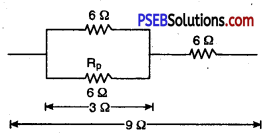∴ $$\frac{1}{6}+\frac{1}{6}$$
= $$\frac{1+1}{6}$$
= $$\frac{1}{3}$$
or Rp = 3 Ω
When this combination is connected in series with third resistor of 6 Ω, it gives a total resistance
(R) = Rp + 6 Ω
= 3Ω + 6Ω
= 9 Ω

(ii) When two resistors each of 6 Ω are connected in series, it gives rise to
Rs = 6 + 6 = 12 Ω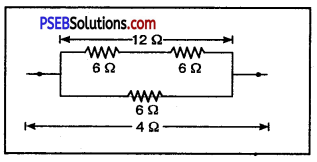This 12 Ω when connected in parallel with 6 Ω gives total resistance Rp’
$$\frac{1}{R_{p}^{\prime}}=\frac{1}{12}+\frac{1}{6}$$
= $$\frac{1+2}{12}$$
= $$\frac{3}{12}$$
= $$\frac{1}{4}$$
Rp’ = 4Ω

Question 12.
Several electric bulbs designed to be used on a 220 V electric supply line are rated 10 W. How many lamps can be connected in parallel with each other across the two wires of 220 V line if the maximum allowable current is 5 A?
Given voltage V = 220 V
Current I = 5A
P = 10 W
∴ Resistance of each bulb, R = $$\frac{\mathrm{V}^{2}}{\mathrm{P}}$$
= $$\frac{(220)^{2}}{10}$$
= $$\frac{220 \times 220}{10}$$
= 4840 Ω

Let ‘n’ be the number of bulbs of 4840 Ω resistance each connected in parallel. Their equivalent resistance Rp is given by ;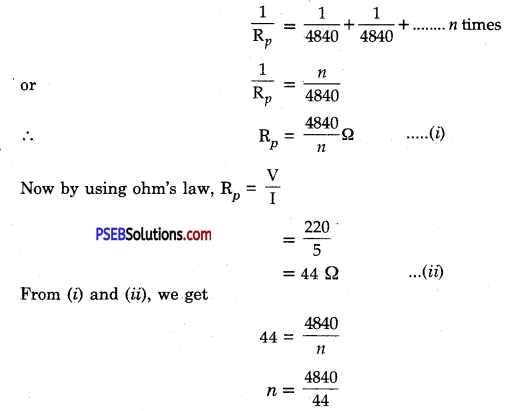∴ n = 110
So, 110 bulbs can be connected in parallel (with each óther across two wires).

Question 13.
A hot plate of an electric oven connected to a 220 V line has two resistance coils A and B, each of 24 Ω resistance, which may be used separately, in series or in parallel. What are currents in three cases?
1. When two coils A and B each of 24 Ω are used separately
R = 24 Ω and V = 220V
∴ Isp = $$\frac{220}{24}$$ = 9.17 A

2. When coils A and B are connected in series :
Resultant Resistance Rs = 24 Ω + 24 Ω = 48 Ω
∴Current Isr = $$\frac{220}{48}$$ = 4.58 A

3. When two coils A and B each of 24 Ω are connected in parallel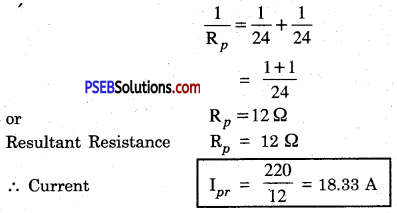Question 14.
Compare the power used in 2 Ω resistor in each of the following circuits :
(i) a 6 V battery in series with 1 Ω and 2 Ω resistors and
Here R1 = 1 Ω ; R2 = 2 Ω
when connected in series the resultant resistance, R = R1 + R2
= 1 Ω + 2 Ω.
= 3 Ω
∴ Current through each resistor and also total current
I = $$\frac{\mathrm{V}}{\mathrm{R}_{s}}$$
= $$\frac{6}{3}$$
= 2A

Power used in 2 Ω resistor P1 = I2 × R
= (2)2 × (2)
= 4 × 2
= 8 W
i.e Power P1 = 8W

(ii) a 4 V battery in parallel with 12 Ω and 2 Ω resistors.
Let 2 Ω and 12 Ω in parallel combination give resultant resistance R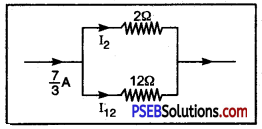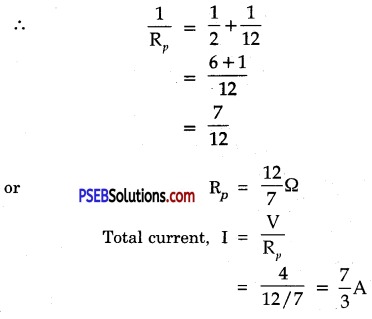Current divides among its parallel resistances in the inverse ratio of resistances.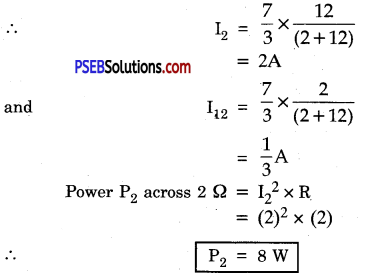It is seen that P1 = P2 = 8W .
The Power consumed by 2 Ω resistor in each case is 8 W.Question 15.
Two lamps, one rated loo W at 220 V and other 60 w at 220 V, are connected in parallel to electric main supply. What current is drawn from the line if the supply voltage is 220 V?
Given Power, P = V2/R :
or R = V2/P
Resistance of loo W lamp R1 = $$\frac{(220)^{2}}{100}$$
= 484 Ω

Resistance of 60 W lamp R2 = $$\frac{(220)^{2}}{60}$$
= 806.7 Ω

Let Rp be the resultant resistance when two lamps are connected in parallel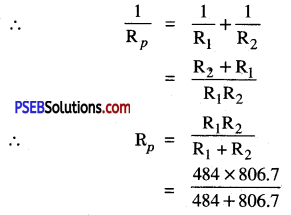Rp = 302.5 Ω
Current drawn from the line, I = $$\frac{\mathrm{V}}{\mathrm{R}_{p}}$$
= $$\frac{220}{302.5}$$
= 0.727A

Question 16.
Which uses more energy, a 250 W TV set for 1 hour or a 1,200 W toaster for 10 minutes?
(i) Energy consumed when TV set of 250W is used for 1 hr. .
E1 = P1 × t2
= 250 W × l h
∴ E1 = 250 Wh

(ii) Energy consumed when 1200 W toaster is used for 10 minutes.
= 1,200 W × $$\frac{10}{60}$$hr
E2 = 200 Wh.
From (i) and (ii) E1 > E2
Therefore, 250 W TV set consumes more energy than a toaster of 1200 W.

Question 17.
An electric heater of resistance 8 Q draws 15 A from service mains for 2 hours. Calculate the rate at which heat is developed in the heater.
Given R = 8 Ω, I = 15A and Time t = 2 hrs.
Rate at which heat is developed in the heater, its electric power,
P = I2 × R
= 152 × 8
= 225 × 8
i.e P = 1,800 W or Js-1
Thus, 1,800 joule of heat is developed per second

Question 18.
Explain the following :
(a) Why is the tungsten used almost exclusively for filament of electric lamps?
Melting point of tungsten is very high and also its resistance is high. Hence tungsten can be heated to very high temperature. Due to its high resistance, large quantity of heat is produced which makes it glow.

(b) Why are the conductors of electric heating devices, such as bread toasters and electric irons, m^e of an alloy rather than pure metal?
Resistivity of alloys is very large as compared to pure metals. Moreover, alloys donot/Oxidise (burn out) easily as compared to pure metals. Therefore, conductors of electric heating devices are made of alloys.

(c) Why is series arrangement not used in domestic circuits?
When electric appliances are connected in series,’ the equivalent resistance will be very large as
Req = R1 + R2 + R3 + R4 + ………. Due to high equivalent resistance huge quantity of heat is produced in the domestic circuit which may result in fire. This is why series arrangement is not used in domestic circuits.

(d) How does the resistance of a wire vary with its area of cross-section?
Resistance of a conductor is inversely proportional to its area cross-section.

(e) Why are copper and aluminium usually employed for electricity transmission?
Copper and aluminium have low resistivity and as such there will be small loss of energy when a certain current flows through them. Therefore, copper and aluminium wires are used for electricity transmission.

Science Guide for Class 10 PSEB Electricity InText Questions and Answers

Question 1.
What is electric circuit?
Electric Circuit: The continuous or closed path of electric current from electric source flowing through different components is called electirc circuit.

Components of Electric Circuit: The main components of electric circuit are :

• Electric source (i.e. battery or cell)
• Conductor
• Switch (or key)
• Any other instrument connected in the circuit.

Question 2.
Define uint of electric current.
S.I unit of electric current is ‘ampere’ which is denoted by ‘A’.

Ampere: When 1 coulomb of charge flows through a conductor in 1 second, then the electric current flowing through conductor is 1 ampere.
∴ 1A = $$\frac{1 \mathrm{C}}{\mathrm{ls}}$$

Question 3.
Calculate the number of electrons that constitute 1 coulomb of charge.
We know, the charge present on 1 electron = 1.6 × 10-19 C
Let ‘n be the number of electrons that constitute 1 coulomb of charge.
∴ n × 1.6 × 10-19 = 1 C
n = $$\frac{1}{1.6 \times 10^{-19}}$$
= $$\frac{1 \times 10^{19}}{1.6}$$
= $$\frac{10}{16}$$ × 1019
= 0. 625 × 1019
= 6.25 × 1018

Question 4.
Name a device that helps to maintain a potential difference across a conductor.
Electric cell or battery is a device that helps to maintain a potential difference across the ends of a conductor. Chemical action within the cell generates the potential difference across the terminals of the cell.

Question 5.
What is meant by saying that the potential difference betwen two points is IV?
The potential difference between two points in a current carrying conductor is said to be 1 V if 1 joule of work is done in moving a charge of one coulomb (1 C) from one point to the other.
i.e 1V = $$\frac{1 \mathrm{~J}}{1 \mathrm{C}}$$
or 1V = 1 JC-1

Question 6.
How much energy is given to each coulomb of charge passing through a 6 V battery?
Energy to be given to 1 C of charge to pass through T V potential difference = 1 J.
Energy to be given to 1C of charge to pass through 6 V battery = 6 J or W = VQ
= 6 volt x 1 coulomb
= 6$$\frac{1 \mathrm{~J}}{1 \mathrm{C}}$$ × 1C
= 6 J.

Question 7.
On what factors does the resistance of a conductor depend?
Or
What is the resistance of a conductor? State the factors on which resistance of a conductor depends?
Resistance of a conductor is the opposition offered by it to the flow of electricity through it.

Factors on which resistance of a conductor depends :
Resistance R of a conductor depends upon :

• length of the conductor. It has been experimentally observed that :
R ∝ l, the length of the wire
• Area of a cross-section of the conductor.
i.e. R ∝ 1/A, where A is the area cross-section of the wire
Combining above, we have :
R ∝$$\frac{l}{\mathrm{~A}}$$
• Nature of material of the conductor.
or R = ρ $$\frac{l}{\mathrm{~A}}$$
where p is a constant called specific resistance or electric resistivity of the material of the conductor.

If l = 1, A = 1, ρ = R. .
i. e., specific resistance (or electric resistivity) of a conductor is the resistance of a wire of unit length and a unit area cross-section. It may be defined as :

Specific resistance of a conductor is the resistance of unit cube of the conductor.

Unit of Specific Resistance ρ : ρ = $$\frac{\mathrm{RA}}{l}$$
= $$\frac{\mathrm{ohm} \mathrm{m}^{2}}{\mathrm{~m}}$$ = ohm m.Question 8.
Will the current flow more easily through a thick wire or a thin wire of the same material when connected to the same source? Why?
We know that the resistance of a wire is inversely proportional to the area. Therefore, the thicker the wire, more is the area and lesser the resistance. Thus, current will flow more easily through a thicker wire than through thin wire of the same material.

Question 9.
Let the resistance of an electrical component remain constant while the potential difference across the two ends of the component decreases to half the former value. What change will occur in the current through it?

• According to Ohm’s law, physical conditions remaining constant, V ∝ I where V is the potential difference across the ends of conductor and I, the current flowing through it.
• When potential difference is halved, the current will also be halved since resistance remain constant.

Question 10.
Why are coils of electric toasters and electric irons made of an alloy rather than that of a pure metal?
Resistivity (specific resistance) of alloys is much higher than pure metals. Moreover, alloys also do not oxidise readily at very high temperatures. The alloys are, therefore, commonly used in making coils of electric heating devices like electric iron, electric toasters etc.

Question 11.
(a) Which among iron and mercury a better conductor?
Resistivity of iron is 10 × 10-8 ohm-m and that of mercury 94 × 10-8 ohm-m, therefore, iron is a better conductor as compared to mercury.

(b) Which material is best conductor?
As silver has low resistivity of 1.60 × 10-8 ohm-m, so silver is the best conductor.

Question 12.
Draw schematic diagram of a circuit consisting of a battery of three cells of 2 V each, a 5 Ω resistor; an 8 Ω resistor and 12 Ω resistor and a plug key, all connected in series.
Schematic diagram of the circuit.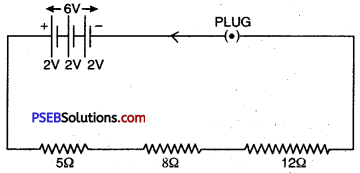Question 13.
Redraw the circuit in above question putting an ammeter to measure the current through the resistors and voltmeter to measure the potential difference across 12 Ω resistor. What would be the reading in the ammeter and the voltmeter?
Modified circuit is as shown in Figure Since 5 Ω, 8 Ω and 12 Ω are connected in series, therefore, total resistance in series : (Rs) = R1 + R2 + R3
= 5 + 8 +12 = 25 Ω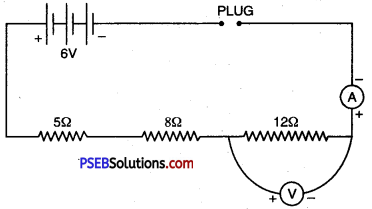Now, Total Current through the circuit, I = $$\frac{\mathrm{V}}{\mathrm{R}}$$
= $$\frac{6}{25}$$ = 0.24 A
∴ Reading of ammeter = 0.24 A
P.D. across 12 Ω i.e., reading of voltmeter
V = I × R
= 0.24 × 12.
V = 2.88 Ω

Question 14.
Judge the equivalent resistance when the following are connected in parallel (a) 1 Ω and 106 Ω ; (b) 1 Ω and 103 Ω and 106 Ω.
(a) Here, R1 = 1 Ω and R2 = 106 Ω
When resistances are connected in parallel.
∴ $$\frac{1}{\mathrm{R}_{p}}=\frac{1}{\mathrm{R}_{1}}+\frac{1}{\mathrm{R}_{2}}$$
= $$\frac{1}{1}+\frac{1}{10^{6}}$$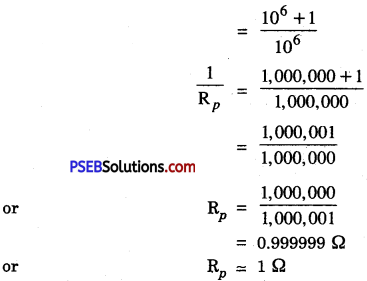(b) Here, R1 = 1 Ω ; R2 = 103 Ω ; R3 = 106
In parallel combination of resistances, $$\frac{1}{\mathrm{R}_{p}}=\frac{1}{\mathrm{R}_{1}}+\frac{1}{\mathrm{R}_{2}}+\frac{1}{\mathrm{R}_{3}}$$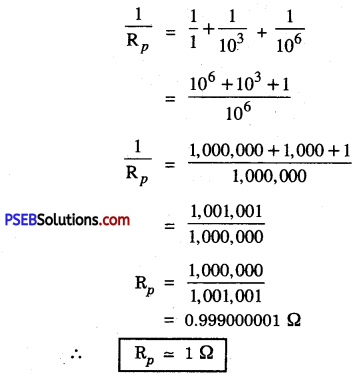Question 15.
An electric lamp of 100 Ω, a toaster of resistance 50 Ω and a water filter of resistance 500 Ω are connected in parallel to a 220 V source. What is the resistance of the electric iron connected to the same source that takes as much current as all the three appliances and what is the current through it?
Combined resistance of 100 Ω, 50 Ω and 500 Ω in parallel i.e., Rp is given by :
$$\frac{1}{\mathrm{R}_{p}}=\frac{1}{100}+\frac{1}{50}+\frac{1}{500}$$
= $$\frac{5+10+1}{500}$$
= $$\frac{16}{500}$$
Rp = $$\frac{500}{16}$$
= 31.25 Ω.
∴ Resistance of electric iron, Rp= 31.25 Ω

Current through electric iron, I = $$\frac{V}{R}$$
= $$\frac{220}{31.25}$$
∴ J = 7.04 A

Question 16.
What are the advantages of connecting electric devices in parallel with the battery instead of connecting them in series?
When connected in parallel, all the electric devices are connected to the same potential difference but they draw different current. Moreover, in parallel arrangement if one electric device fails to work, the other devices are not affected. But when connected in series, the potential is divided between the different devices. In case, if one device is switched off or slops working, the other devices also do not work.

Question 4.
How can three resistors of resistance 2 Ω, 3 Ω and 6 Ω be connected to give a total resistance of (a) 4 Ω ; (6) 1 Ω?
(a) When all the three resistors are connected in series, the total resistance will be equal to the sum of all the three resistances.
i.e. Rs = 2Ω + 3Ω + 6Ω
= 11Ω this arrangement is rejected.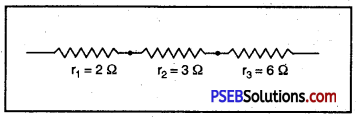(a)
Therefore, when 3 Ω and 6 Ω are in parallel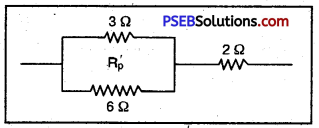(b)
Rp’ = 2 Ω. It is in series with 2 Ω.
∴ Total resistance when 3 Ω, 6 Ω in parallel are in series with 2 Ω is = Rp’ + 2 = 2 + 2 = 4Qas given in Figure (b)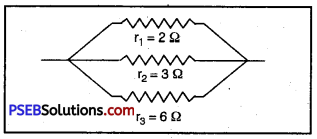(c)
If all the three resistances are in parallel, then
$$\frac{1}{\mathrm{R}_{p}}=\frac{1}{2}+\frac{1}{3}+\frac{1}{6}$$
= $$\frac{3+2+1}{6}$$
= $$\frac{6}{6}$$
Rp = 1 ΩQuestion 17.
What is the (a) highest; (b) lowest total resistance that can be secured by combination of four coils of resistances 4 Ω, 8 Ω, 12 Ω, 24 Ω?
(a) Total resistance will be maximum when all the four resistances are connected in series.
Let Rs be the total resistance in series combination
∴ Rs =R1+ R2 + R3 + R4
= 4Ω + 8Ω + 12Ω + 24Ω
∴ Highest resistance = 48 Ω

(b) Total resistance will be lowest when all the four given resistance are connected in parallel.
Let Rp be the total resistance in parallel combination,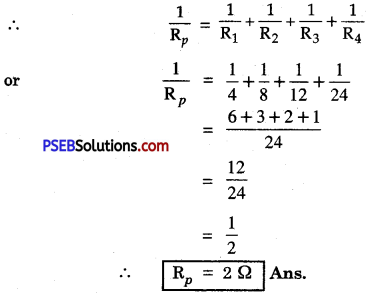Question 18.
Why does the cord carrying an electric current does not glow while heating element does?
Heating effect = 12R where I is the current flowing and R is the resistance.
Current I is the same both in cord and in heating element.

Resistance R of the cord is negligible since it is made of copper (which has very less resistivity) while heating element is made of nichrome whose resistivity is 6,000 times more than copper. Nichrome gets heated up much more than copper. Therefore, heating element glows.

Question 19.
Compute the heat generated while transferring 96,000 C of charge in one hour through a potential difference of 50 V.
Here, Quantity of charge (q) = 96000 C transferred in 1 hr.
Potential difference = 50 V
Work done to transfer Q coulomb of charge through potential difference W = VQ joule
W = 50 × 96,000
= 48,00,000 J
W = 4.8 × 106 J
∴ Heat produced = Work done in transferring the charge = 4.8 × 106 J

Question 20.
An electric iron of resistance 20 Ω takes a current of 5A. Calculate the heat developed in 30 s.
Here, Resistance, R = 20 Ω ; I = 5 A ; t = 30 s.
We know, heat produced H = I2 Rt
= 52 × 20 × 30
H = 15,000 J

Question 21.
What determines the rate at which the energy is delivered by a current?
Rate at which energy is delivered by the current is called power. It is measured in watt or in kilowatt.

Question 22.
An electric motor takes 5 A from a 220 V line. Determine the power of the motor and the energy consumed in 2 h.